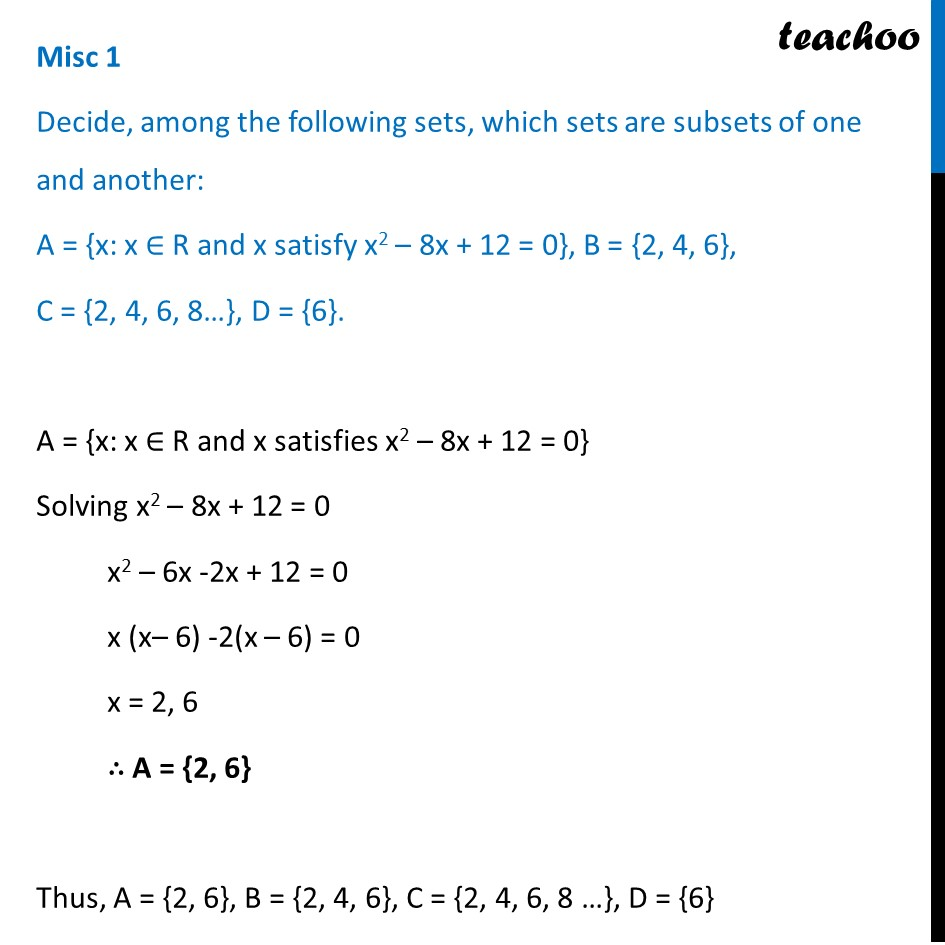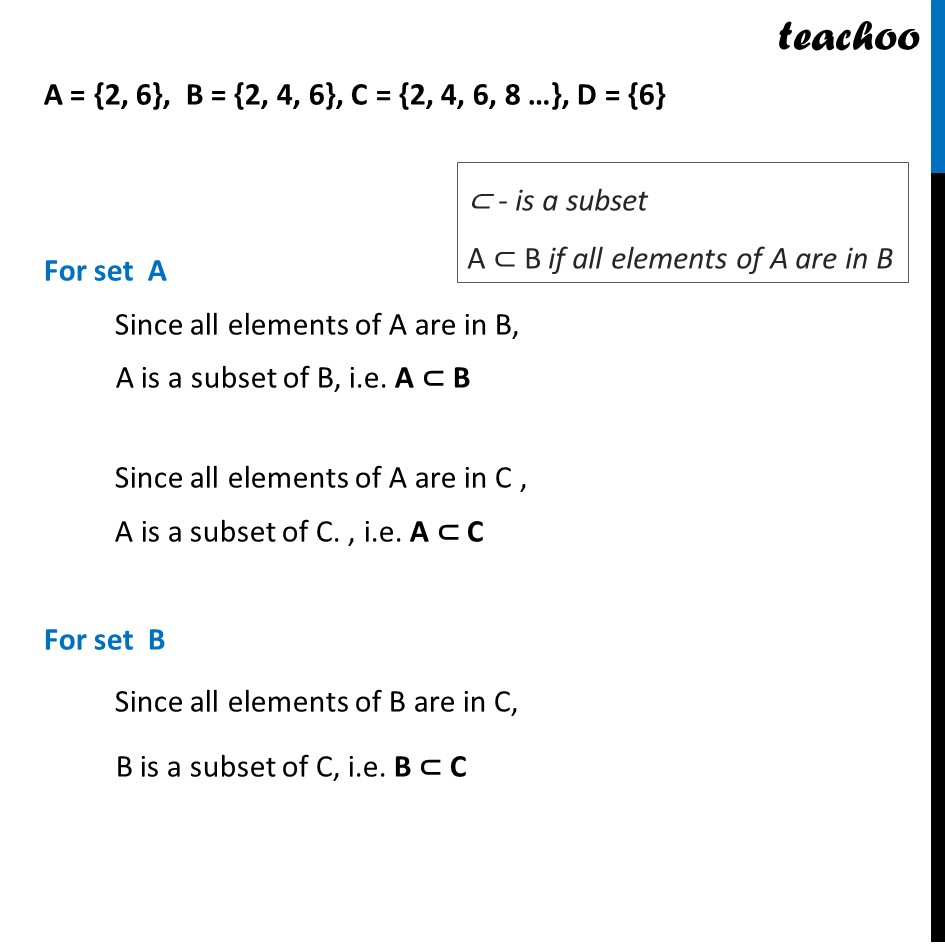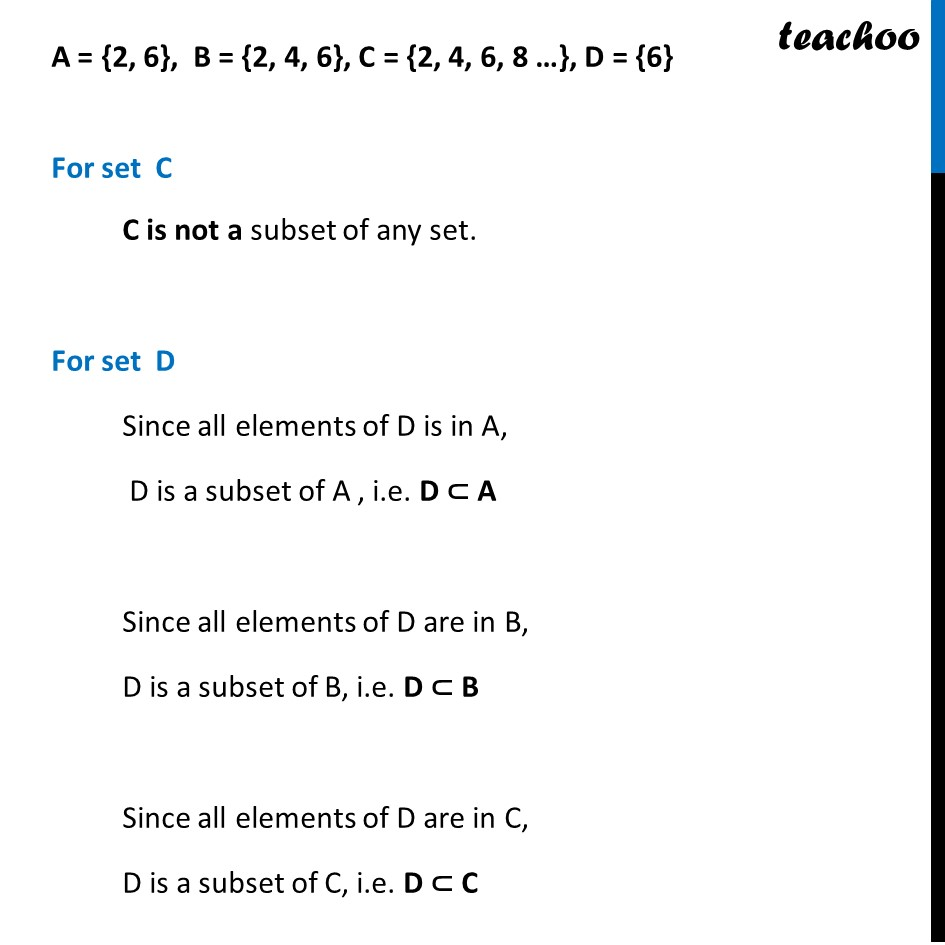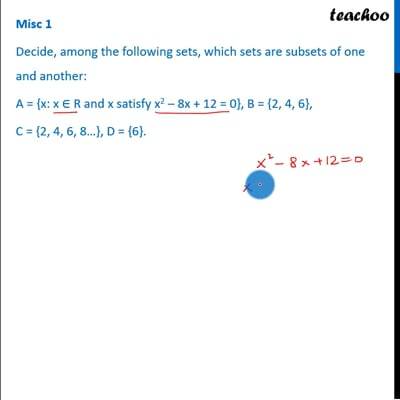Miscellaneous

Chapter 1 Class 11 Sets (Term 1)
Serial order wiseThis video is only available for Teachoo black users

### Transcript

Misc 1Misc 1 Decide, among the following sets, which sets are subsets of one and another: A = {x: x ∈ R and x satisfy x2 – 8x + 12 = 0}, B = {2, 4, 6}, C = {2, 4, 6, 8…}, D = {6}. A = {x: x ∈ R and x satisfies x2 – 8x + 12 = 0} Solving x2 – 8x + 12 = 0 x2 – 6x -2x + 12 = 0 x (x– 6) -2(x – 6) = 0 x = 2, 6 ∴ A = {2, 6} Thus, A = {2, 6}, B = {2, 4, 6}, C = {2, 4, 6, 8 …}, D = {6} A = {2, 6}, B = {2, 4, 6}, C = {2, 4, 6, 8 …}, D = {6} For set A Since all elements of A are in B, A is a subset of B, i.e. A ⊂ B Since all elements of A are in C , A is a subset of C. , i.e. A ⊂ C For set B Since all elements of B are in C, B is a subset of C, i.e. B ⊂ C A = {2, 6}, B = {2, 4, 6}, C = {2, 4, 6, 8 …}, D = {6} For set C C is not a subset of any set. For set D Since all elements of D is in A, D is a subset of A , i.e. D ⊂ A Since all elements of D are in B, D is a subset of B, i.e. D ⊂ B Since all elements of D are in C, D is a subset of C, i.e. D ⊂ C Decide, among the following sets, which sets are subsets of one and another: A = {x: x ∈ R and x satisfy x2 – 8x + 12 = 0}, B = {2, 4, 6}, C = {2, 4, 6, 8…}, D = {6}. A = {x: x ∈ R and x satisfies x2 – 8x + 12 = 0} Solving x2 – 8x + 12 = 0 x2 – 6x -2x + 12 = 0 x (x– 6) -2(x – 6) = 0 x = 2, 6 ∴ A = {2, 6} Thus, A = {2, 6}, B = {2, 4, 6}, C = {2, 4, 6, 8 …}, D = {6} A = {2, 6}, B = {2, 4, 6}, C = {2, 4, 6, 8 …}, D = {6} A Since all elements of A are in B, A is a subset of B, i.e. A ⊂ B Since all elements of A are in C , A is a subset of C. , i.e. A ⊂ C B Since all elements of B are in C, B is a subset of C, i.e. B ⊂ C ⊂ - is a subset A ⊂ B if all elements of A are in B A = {2, 6}, B = {2, 4, 6}, C = {2, 4, 6, 8 …}, D = {6} C C is not a subset of any set. D Since all elements of D is in A, D is a subset of A , i.e. D ⊂ A Since all elements of D are in B, D is a subset of B, i.e. D ⊂ B Since all elements of D are in C, D is a subset of C, i.e. D ⊂ C